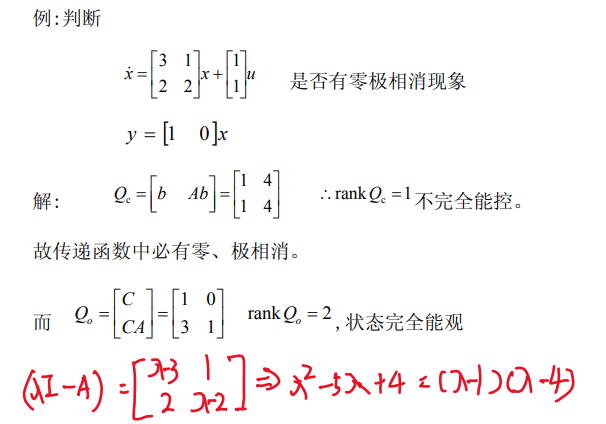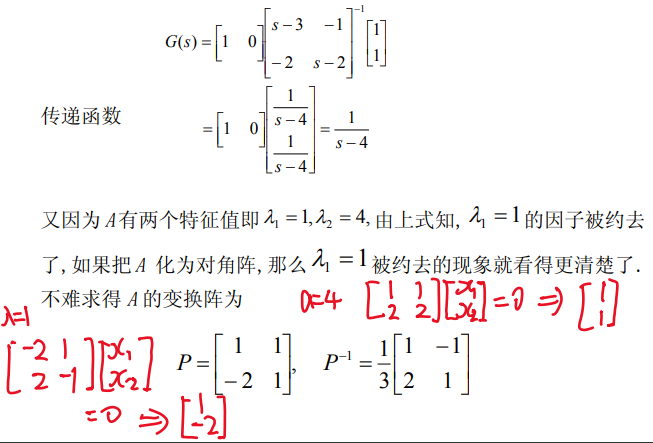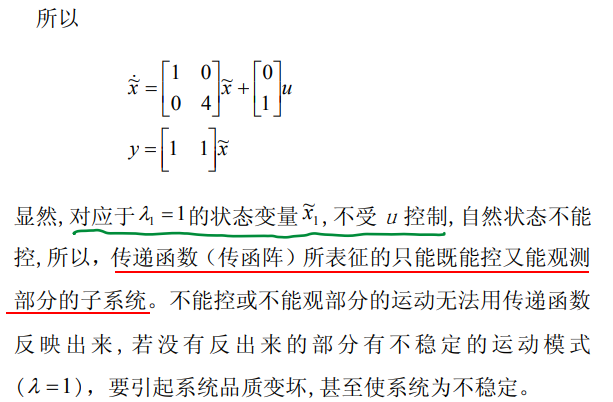# 1. 线性系统的能控性和能观性

## 1.1. 例子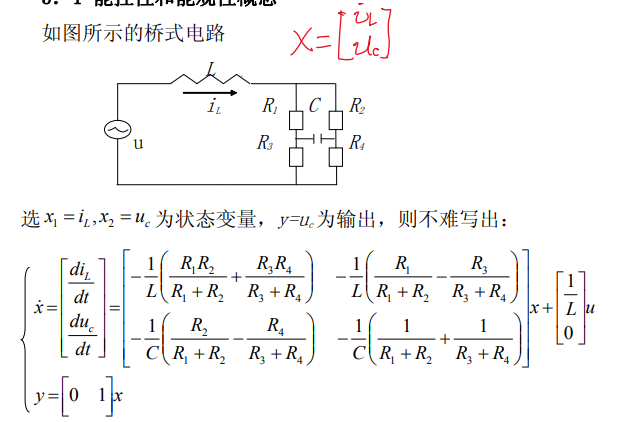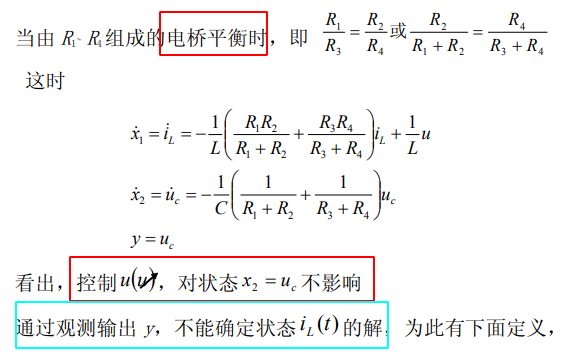• $$x2=u_c$$不能控
• $$x_1=i_L$$不能观

# 2. 定义

## 2.1. 状态能控性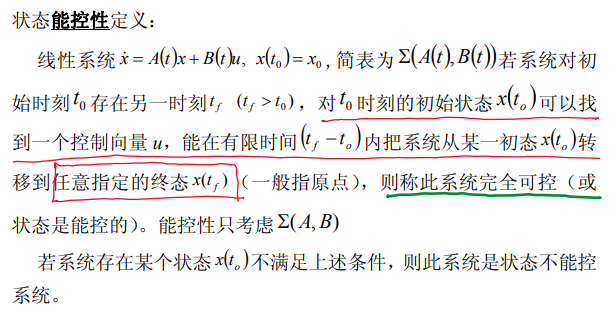## 2.2. 状态能观性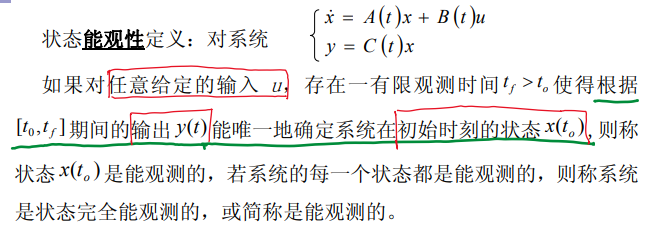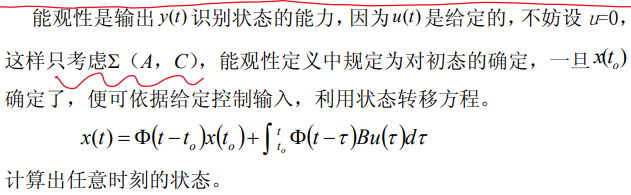# 3. 线性连续时间系统能控性判据

## 3.1. 线性定常系统能控性判据

\left \{ \begin{aligned} &\dot{x}=Ax+Bu \\ &x(0)=x_0 \end{aligned} \right.

### 3.1.1. 格拉姆矩阵判据(Gram)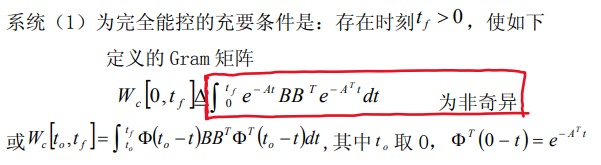• 格拉姆矩阵非奇异

### 3.1.2. 秩判据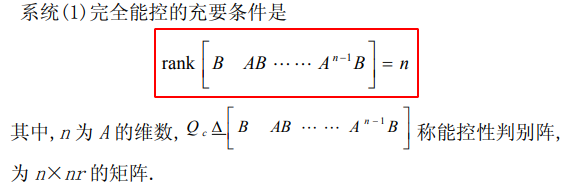#### 3.1.2.1. 例子1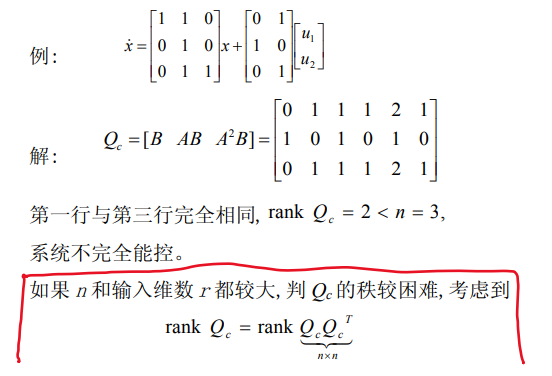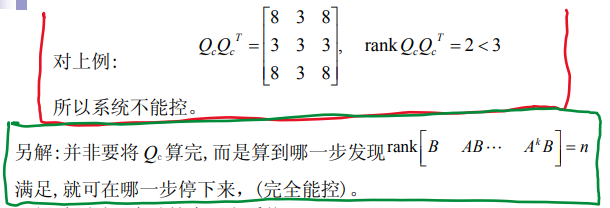#### 3.1.2.2. 例子2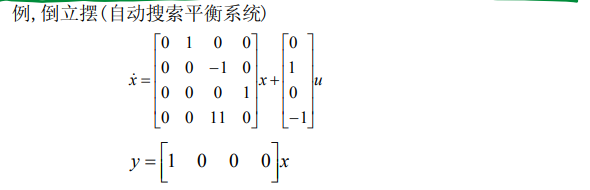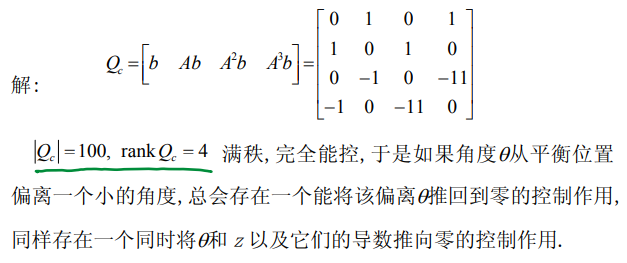### 3.1.3. PBH秩判据(Popov-Belevitch-Hautus)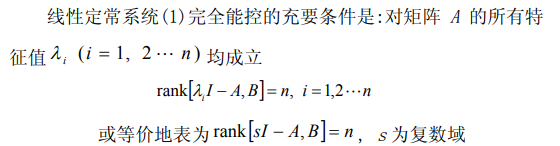#### 3.1.3.1. 例子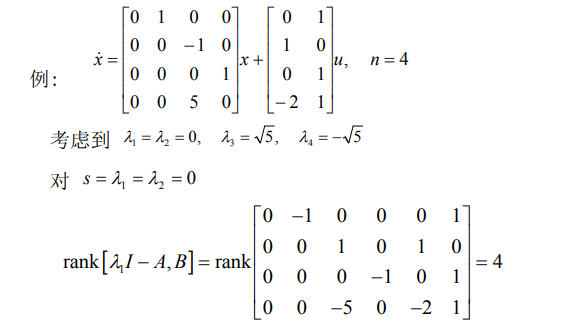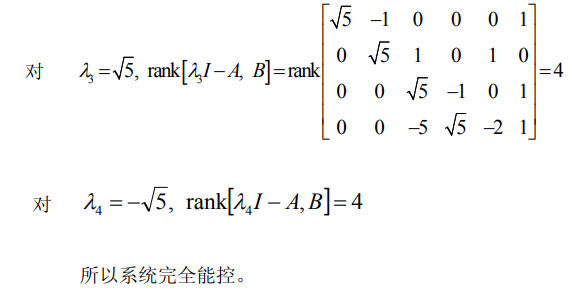### 3.1.4. 约当规范形判据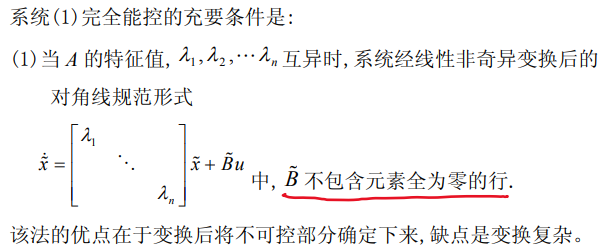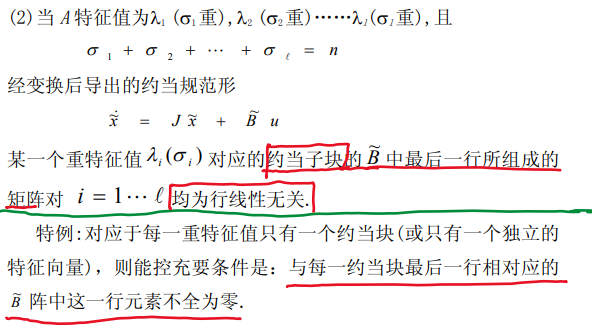#### 3.1.4.1. 例子1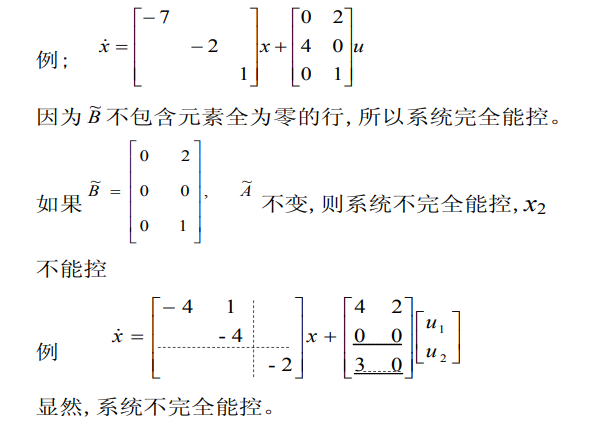#### 3.1.4.2. 例子2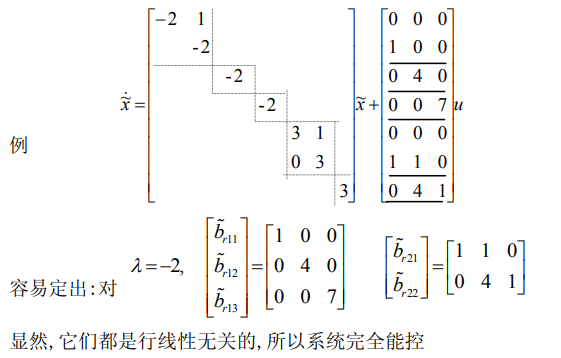## 3.2. 线性时变系统能观性判据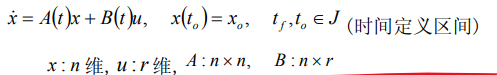### 3.2.1. 格拉姆矩阵判据(Gram)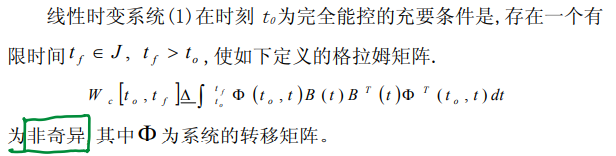### 3.2.2. 秩判据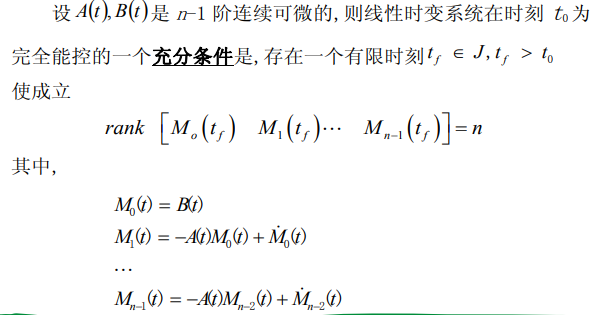#### 3.2.2.1. 例子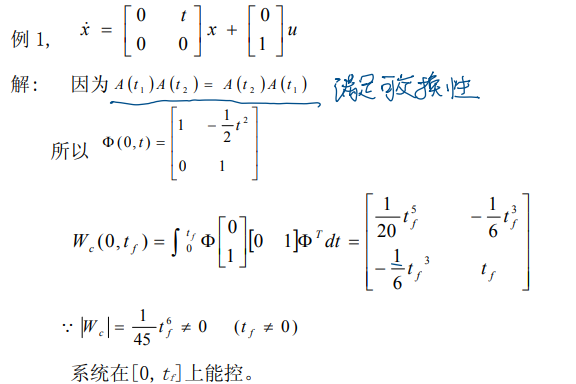# 4. 线性连续时间系统的能观性判据

## 4.1. 线性定常系统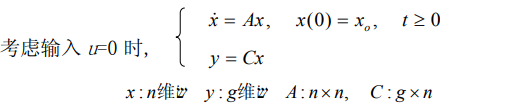### 4.1.1. 格拉姆矩阵判据(Gram)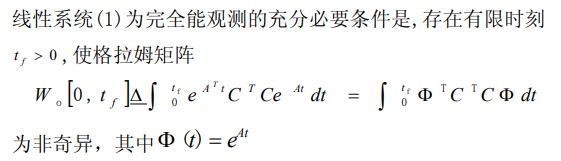### 4.1.2. 秩判据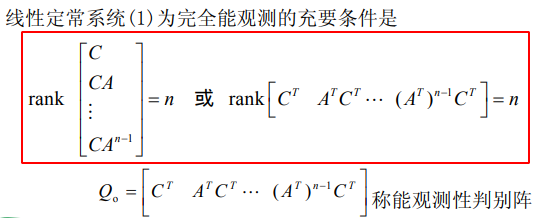### 4.1.3. PBH秩判据(Popov-Belevitch-Hautus)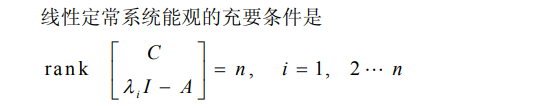### 4.1.4. 约当规范形判据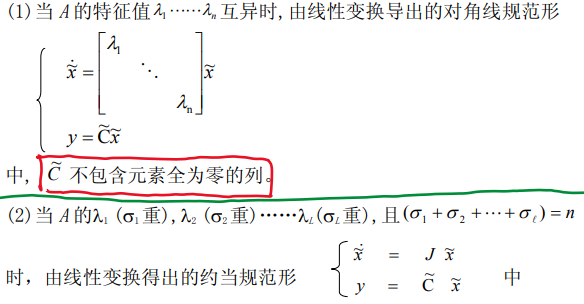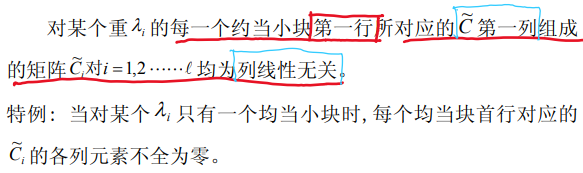#### 4.1.4.1. 例子1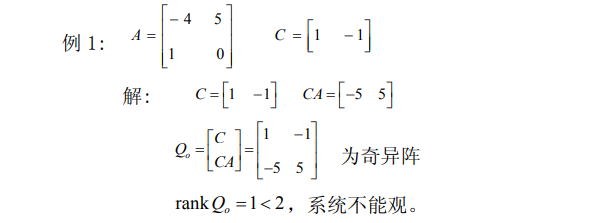#### 4.1.4.2. 例子2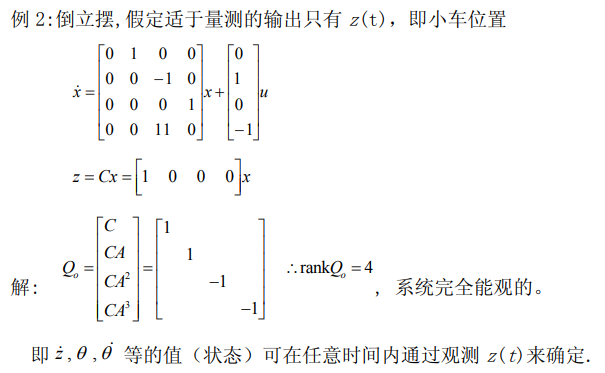#### 4.1.4.3. 例子3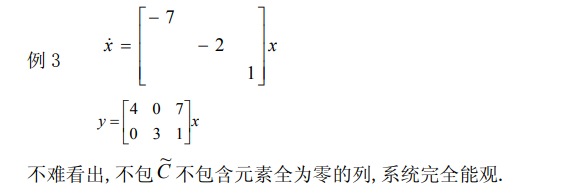#### 4.1.4.4. 例子4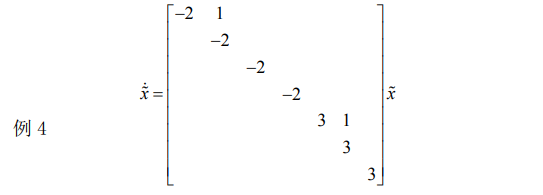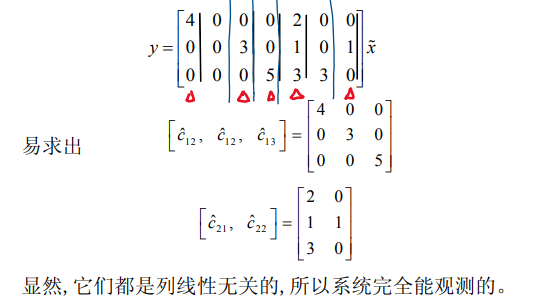#### 4.1.4.5. 例子5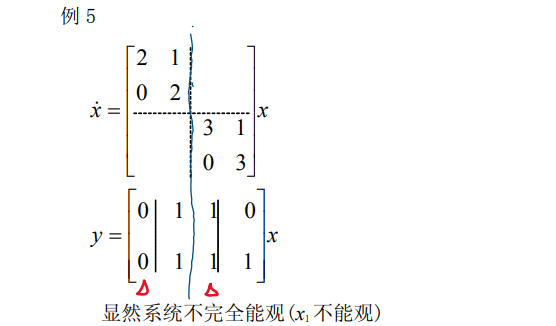## 4.2. 线性时变系统能观性判据

\left \{ \begin{aligned} &\dot{x}=A(t)x , x(t_0)=x_0, t,t_0 \in J \\ &y=C(t)x \end{aligned} \right.

### 4.2.1. 格拉姆矩阵判据(Gram)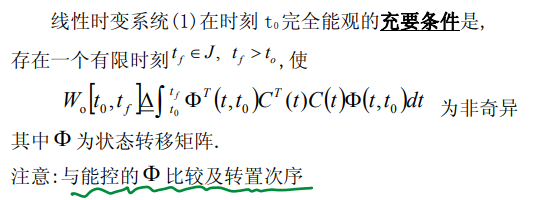### 4.2.2. 秩判据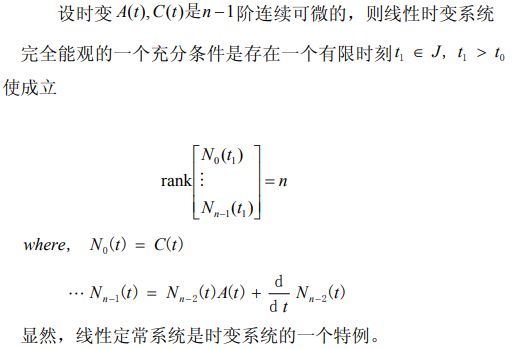# 5. 线性离散系统的能控性和能观性判据

## 5.1. 离散系统能控性

### 5.1.1. 线性时变离散系统——格拉姆矩阵判据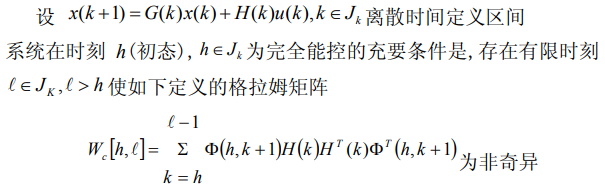### 5.1.2. 线性定常离散系统——秩判据## 5.2. 离散系统能观性

\left \{ \begin{aligned} &x(k+1)=G(k)x(k) \\ &y(k)=C(k)x(k) \end{aligned} \right.

### 5.2.1. 线性时变离散系统——格拉姆矩阵判据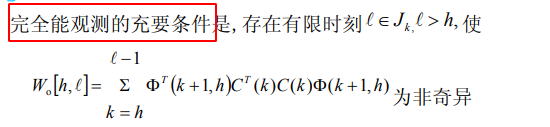### 5.2.2. 线性定常离散系统——秩判据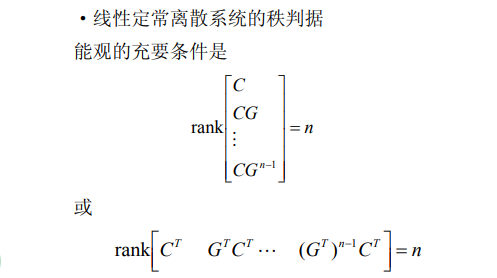## 5.3. 统离散化后保持能控和能观的条件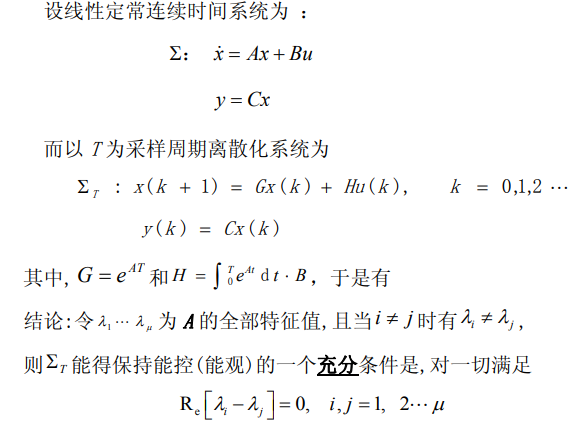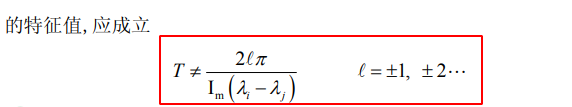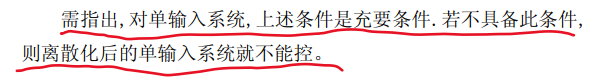### 5.3.1. 例子1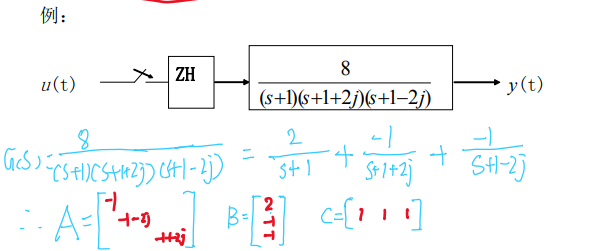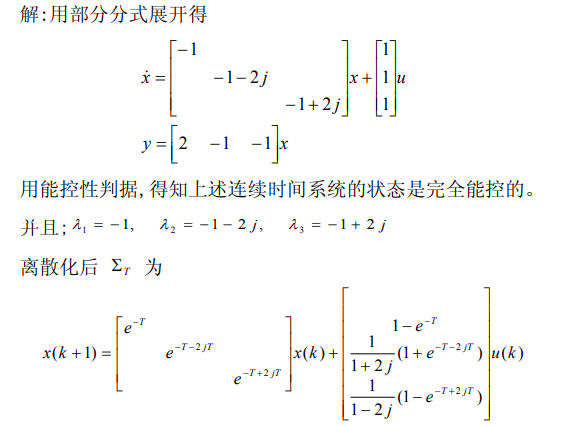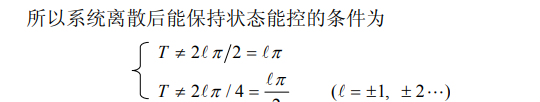### 5.3.2. 例子2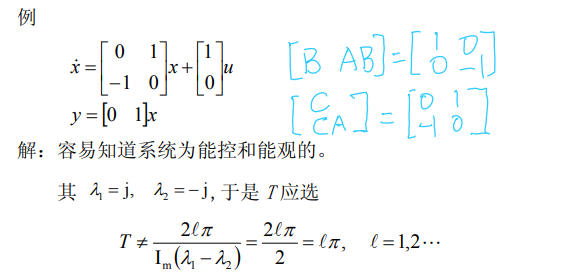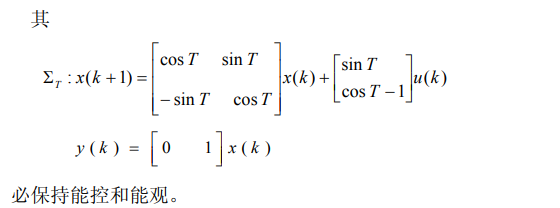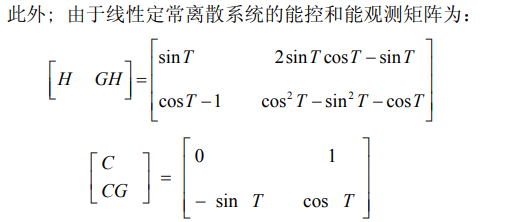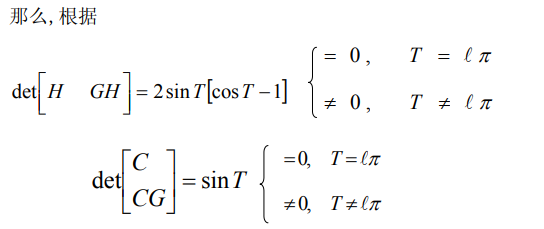# 6. 线性系统能控性与能观测性的对偶关系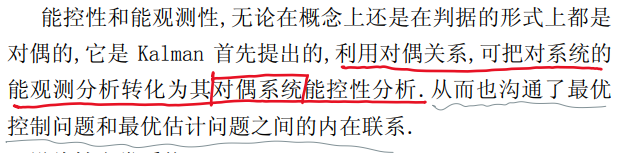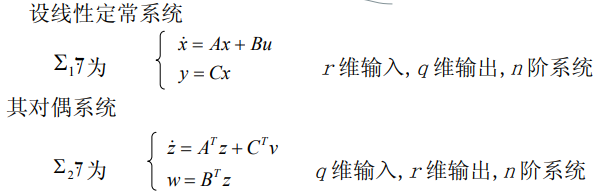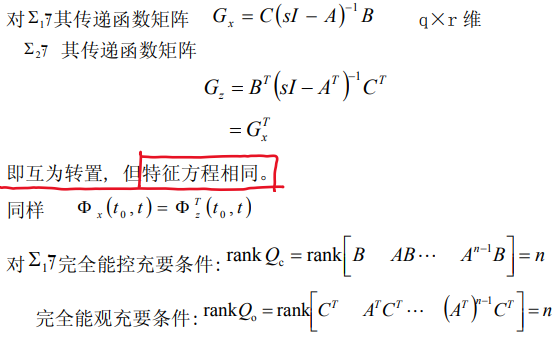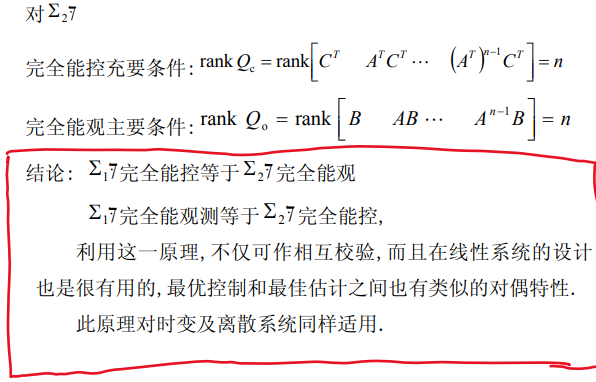# 7. 能控标准形和能观测标准形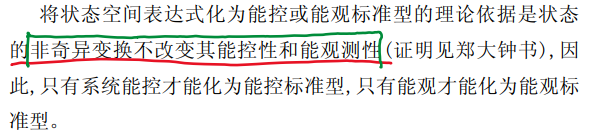## 7.1. 能控规范型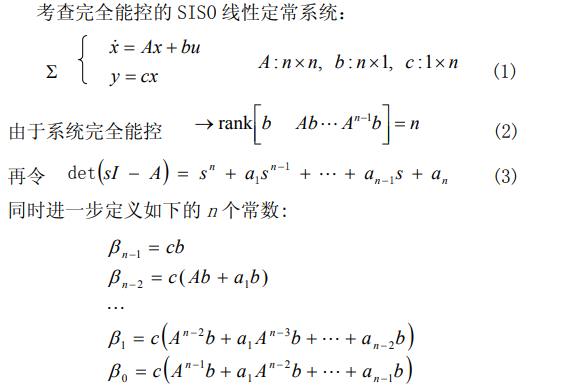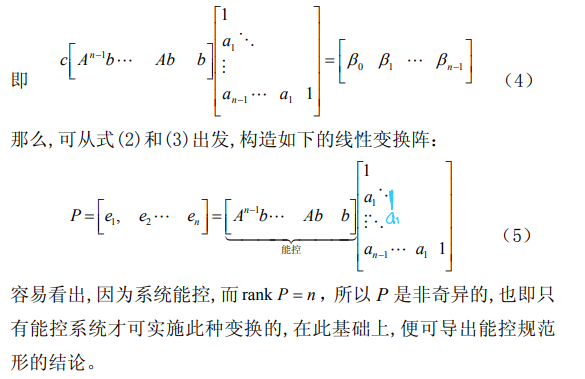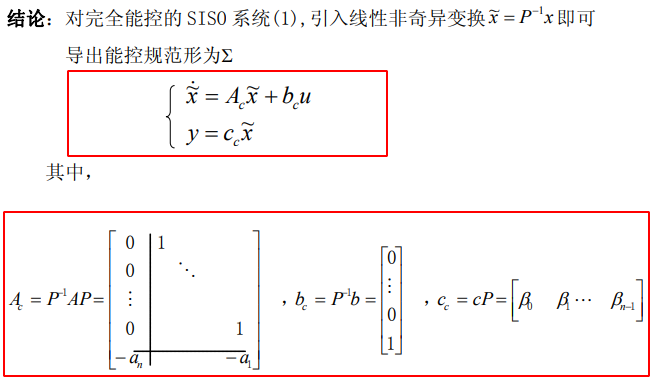### 7.1.1. 例子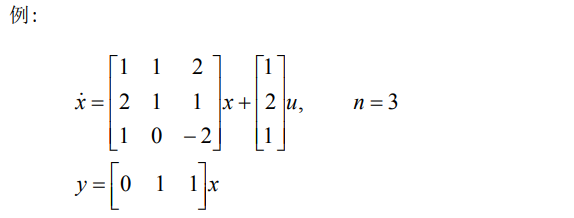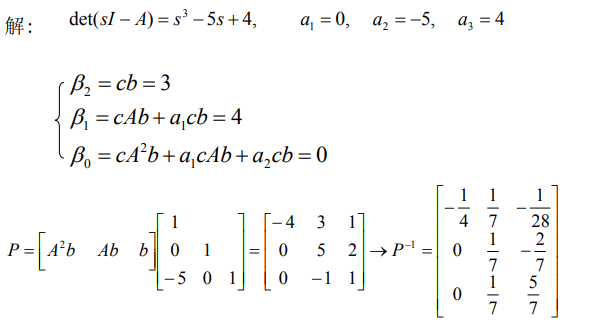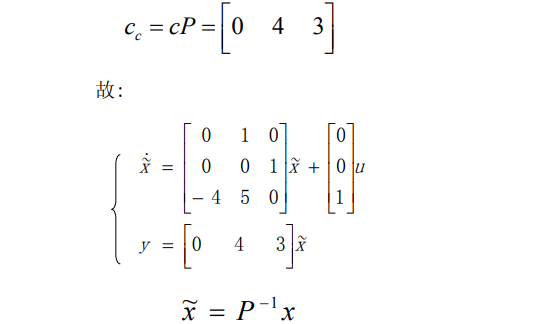## 7.2. 能观规范型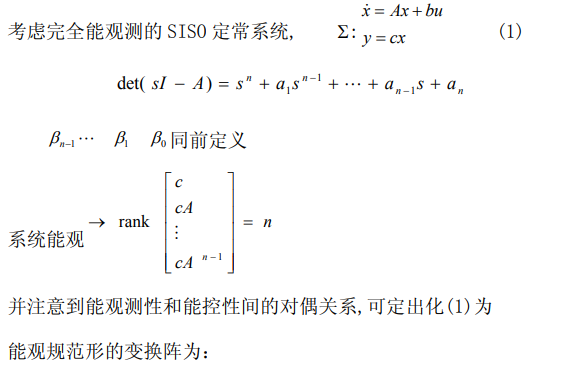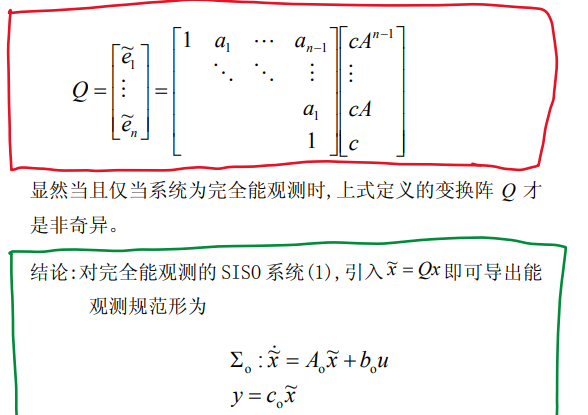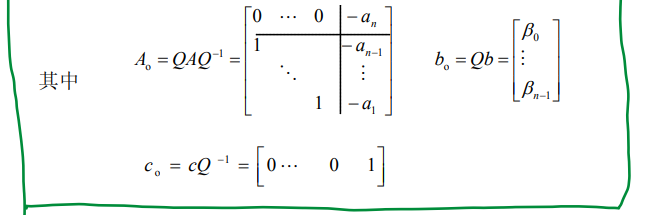### 7.2.1. 例子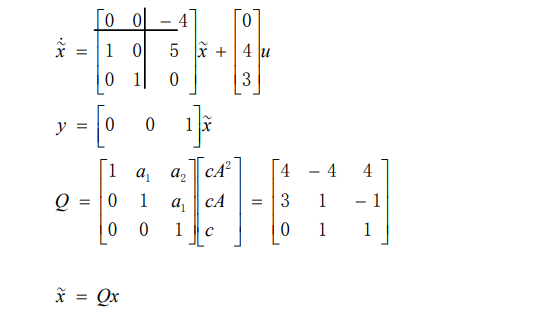# 8. 系统的能控性、能观测性和传递函数阵的关系

## 8.1. 系统的结构分解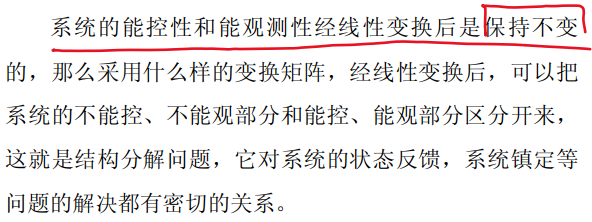### 8.1.1. 按能控性分解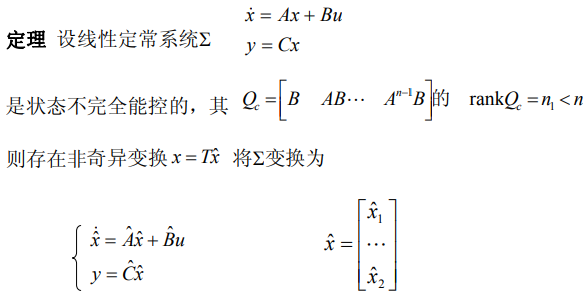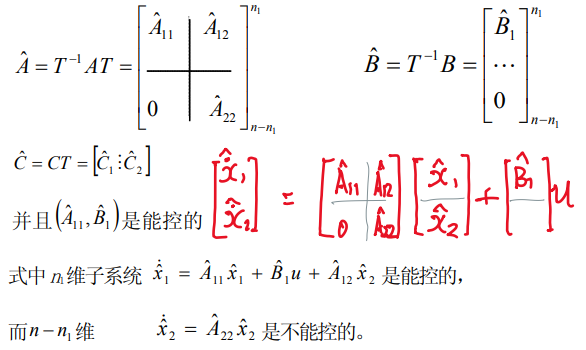#### 8.1.1.1. 非奇异变换矩阵T的选取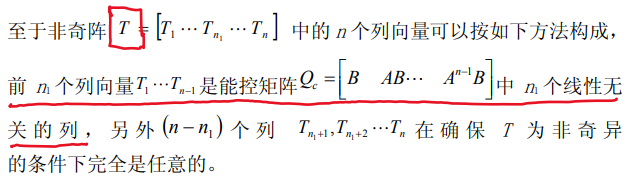#### 8.1.1.2. 例子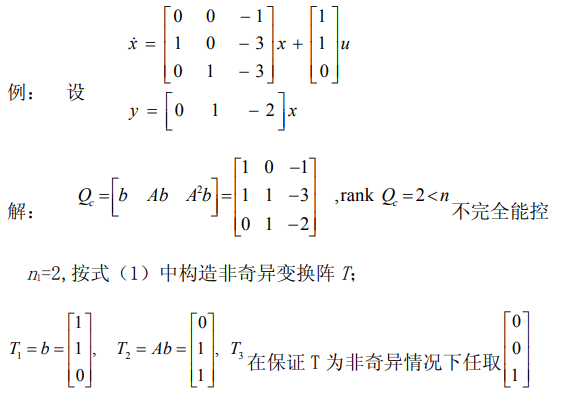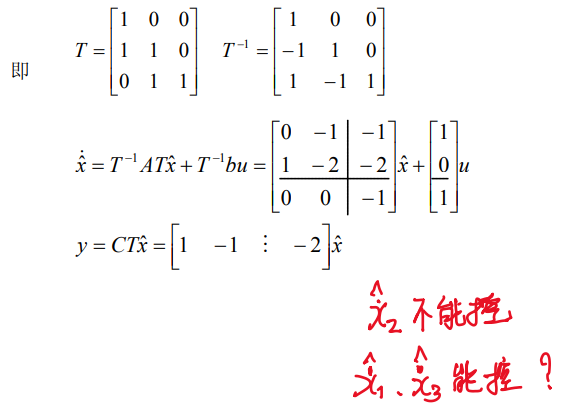### 8.1.2. 按能观性分解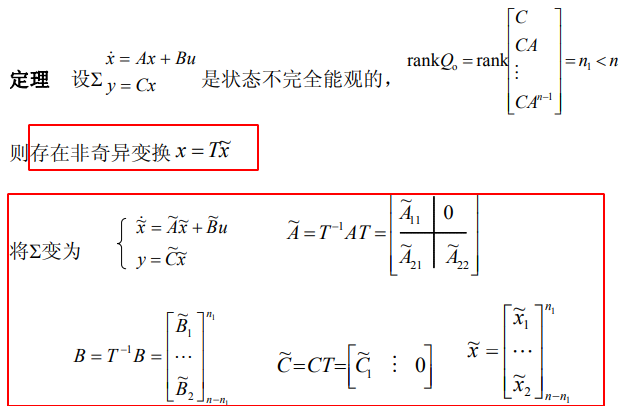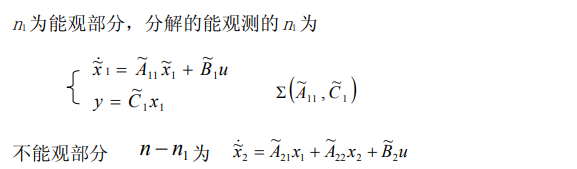#### 8.1.2.1. 非奇异变换矩阵T的选取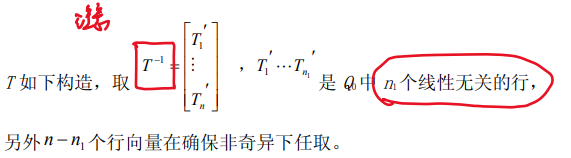#### 8.1.2.2. 例子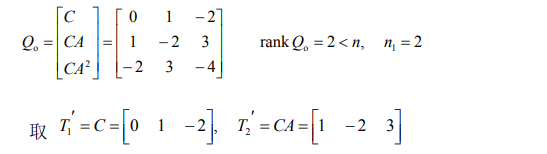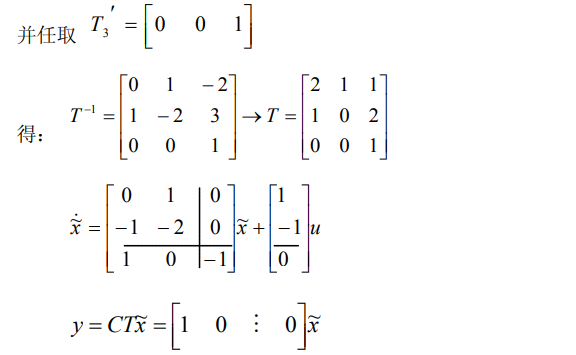### 8.1.3. 规范分解定理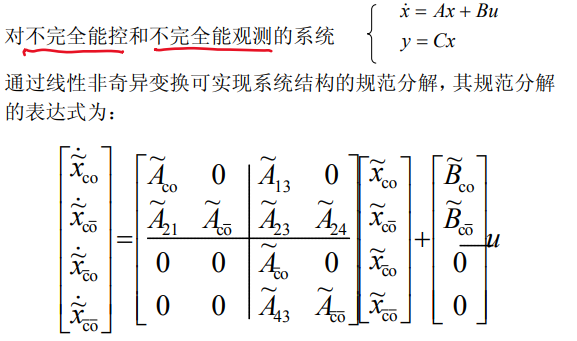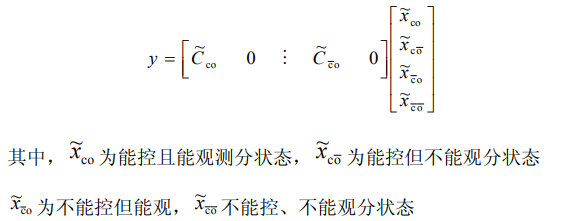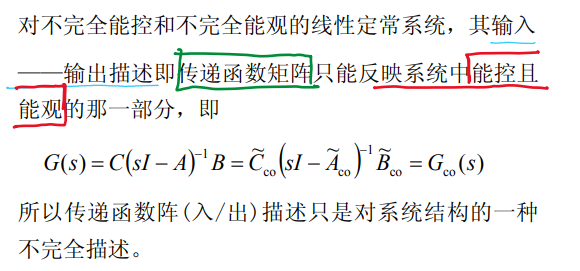#### 8.1.3.1. 传递函数矩阵的最小多项式表示形式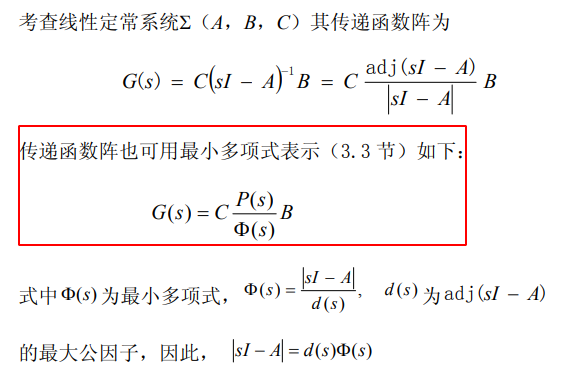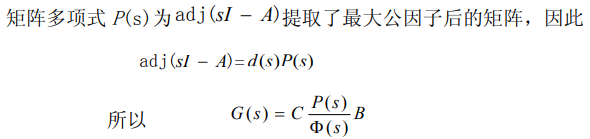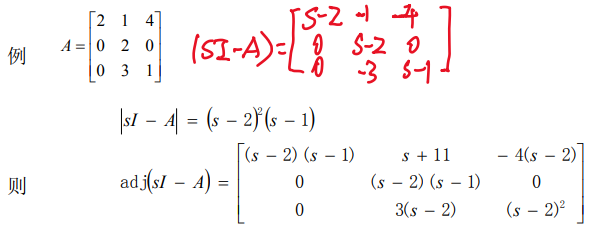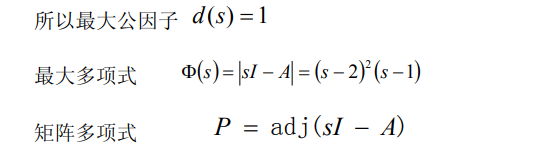### 8.1.4. 传递函数中零、极点相消定理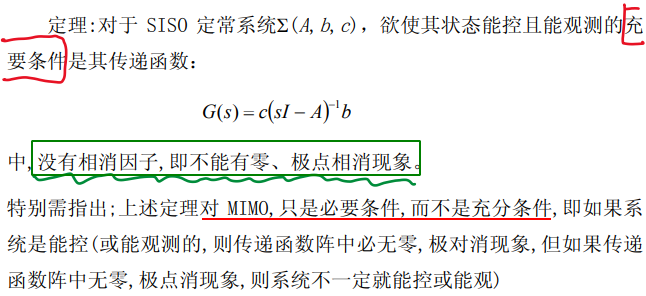#### 8.1.4.1. 例子